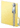Psychometric Toolbox
Norms

Description

Function Scoring and Norming -> linear and nonlinear score transformations and normative tables.

The Scoring and Norming function (a) provides the descriptive statistics of the raw test scores, (b) transforms the individual raw scores, and (c) creates the test normative table.

There are two computing options, explicitly labelled as step 2 and 3 (step 1 is importing the file), because the order of the process is important in this macro: (a) Step 2: Individual transformation, and (b) Step 3: Normative table, which generates the normative table of the instrument.

There is an option for saving all the individual transformed scores.

The output is organized in two parts. The first part, which is obtained by clicking on “individual transformation”, linearly transforms the raw scores of each individual. The raw scores are obtained from the item set which is specified by the user (the default is the whole test), and the possible transformed scores are: standard or typical (0,1), T (50,10), IQ (100, 15) and stanine (5,2) (linear transformations) and Percentile ranks (nonlinear transformations). In addition to the transformed score, the macro provides the corresponding confidence interval and the percentile band at the confidence level specified by the user. The scores, both raw and transformed, can be saved in a text file by clicking on “save individual transformation”.

The second part of the output is obtained by clicking on “normative table”. Three pieces of information are provided: (a) the descriptive statistics of the raw scores together with the corresponding histogram, (b) the frequency table, and (c) the normative table which uses both linear (standard, T, IQ or Stanine) and nonlinear (percentile) transformations. Tables (b) and (c) can be non-grouped or grouped at the user’s request. The non-grouped table uses all the observed scores, and is recommended for short tests which have a limited range of values. Grouped frequency tables are preferable when the range of raw score values is large.

Download example data file:Scoring_and_Norming.zip